# Texas Go Math Grade 3 Lesson 5.3 Answer Key Combine Place Values to Subtract

Refer to our Texas Go Math Grade 3 Answer Key Pdf to score good marks in the exams. Test yourself by practicing the problems from Texas Go Math Grade 3 Lesson 5.3 Answer Key Combine Place Values to Subtract.

## Texas Go Math Grade 3 Lesson 5.3 Answer Key Combine Place Values to Subtract

Unlock the Problem

Elena collected 431 bottles for recycling. Pete collected 227 fewer bottles than Elena. How many bottles did Pete collect?

What do you need to find?
Explanation:
Elena collected 431 bottles for recycling.
Pete collected 227 fewer bottles than Elena.
Number of bottles collected by Pete
431 – 227 = 204

Circle the numbers you need to use.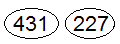Explanation:
Numbers that are used to circle are Elena collected bottles and Pete collected bottles.

Combine place values to find the difference.

A. Subtract. 431 – 227
Estimate. 400 – 200 = ________
STEP 1: Look at the ones place. Since 7 > 1, combine place values. Combine the tens and ones places. There are 31 ones and 27 ones. Subtract the ones. Write 0 for the tens.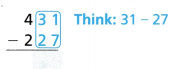Estimate. 400 – 200 = 200

STEP 2:
Subtract the hundreds.
So, Pete collected _________ bottles.
Since ___________ is close to the estimate of _________, the answer is reasonable.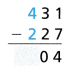So, Pete collected 204 bottles.
Since 204 is close to the estimate of 200, the answer is reasonable.
Explanation:
To find a value that is close enough to the right answer,
usually with some thought or calculation involved. is called estimate.
So, the estimate of 204 is 200.

Math Talk
Mathematical Processes

Explain in why there is a zero in the tens place.
Regrouping the numbers for subtracting the higher number from smaller number

B. Subtract. 500 – 173
Estimate. 500 – 175 = ___________
STEP 1:
Look at the ones and tens places. Since 3 > 0 and 7 > 0, combine the hundreds and tens. There are 50 tens. Regroup 50 tens as 49 tens 10 ones. Subtract the ones.STEP 2:
Subtract the tens.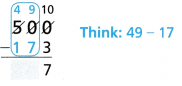So, 500 – 173 __________.
500 – 173 = 327

Math Talk
Mathematical Processes

Explain why you combined hundreds and tens.
Yes, we need to regroup.
Explanation:
Sometimes we need to regroup the hundreds and the tens.
For example,
352 – 185 = 167
Place value disks can help us figure it out.
Subtract ones from ones first.
But we can’t take away five ones,
We only have 2 ones,
So, let’s regroup.

Share and Show

Find 851 – 448 in two ways.

Estimate. 850 – 450 = _________
Estimate. 850 – 450 = 400
450 + 400 = 850

Question 1.
Use place value.Estimate: 400
Explanation: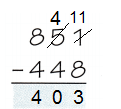Question 2.
Combine place values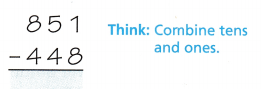Estimate: 400Question 3.
Combine place values to find 406 – 274.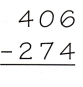Think: Subtract the ones. Then combine the hundreds and tens places.
Estimate: 410
Explanation:subtract first at once place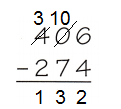Then combine the hundreds and tens places
274 + 132 = 406

Math Talk
Mathematical Processes

Explain how to combine place values
Instead of borrowing or regrouping the number for subtracting,
one can combine the place values.
Explanation:
To combine the place values first combine the tens and ones place.
Then subtract to find the difference.

Estimate. Then find the difference.

Question 4.
Estimate: _______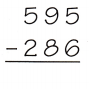Estimate: 300
Explanation:
600 – 300 = 300Since 309 is close to the estimate of 300, the answer is reasonable.

Question 5.Estimate: __________
Explanation:
700 – 500 = 200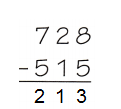Since 213 is close to the estimate of 200, the answer is reasonable.

Question 6.
Estimate: _________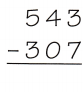Estimate: 230
Explanation:
540 – 310 = 230Since 236 is close to the estimate of 230, the answer is reasonable.

Question 7.
Estimate: ____________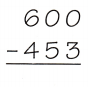Estimate : 150
Explanation:
600 – 450 = 150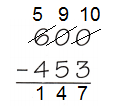Since 147 is close to the estimate of 150, the answer is reasonable.

Problem Solving

Practice: Copy and Solve Estimate. Then solve.

Question 8.
457 – 364
Explanation:
To find a value that is close enough to the right answer is called estimate.
Estimate: 100
457 – 364 = 93
460 – 360 = 100
Since  93 is close to the estimate of 100 the answer is reasonable.

Question 9.
652 – 341
Explanation:
To find a value that is close enough to the right answer is called estimate.
652 – 341 = 311
Estimate:
650 – 340 = 310
Since 311 is close to the estimate of 310, the answer is reasonable.

Question 10.
700 – 648
Explanation:
To find a value that is close enough to the right answer is called estimate.
700 – 648 = 52
700 – 650 = 50
Since 52 is close to the estimate of 50, the answer is reasonable.

Question 11.
963 – 256
Explanation:
To find a value that is close enough to the right answer is called estimate.
963 – 256 = 707
Estimate:
960 – 250 = 710

Question 12.
When does the combine place values strategy make it easier to find the difference? Explain.

Problem Solving

Use Tools Use the table for 13-18.Question 13.
The table shows the heights of some roller coasters in the United States. How much taller is Kingda Ka than Titan?
211 feet
Explanation:
Height of Kingda ka = 456
Height of Titan = 245
The difference between both
456 – 245 = 211

Question 14.
How many feet shorter is Intimidator 305 than Top Thrill Dragster?
115 feet
Explanation:
Height of Top Thrill Dragster = 420
Height of Intimidator = 305
number of feet Intimidator shorter
420 – 305 = 115

Question 15.
Which roller coaster is 175 feet taller than the Titan?
Top Thrill Dragster
Explanation:
Height of Titan = 245
Combine both the heights
245 + 175 = 420
So, Top Thrill Dragster is taller than Titan.

Question 16.
Multi-Step How much shorter is Top Thrill Dragster than the heights of Titan and Kingda Ka together?
281 feet
Explanation:
Height of Titan  = 245
Height of Kingda ka = 456
Height of Top Thrill Dragster = 420
245 + 456 = 701 feet
701 – 420 = 281

Question 17.
H.O.T. What if a roller coaster was 500 feet tall? Which roller coaster would be 195 feet shorter?Intimidator 305
Explanation:
Suppose the height of roller coaster = 500
If the roller coaster is less then 195, then Intimidator is shorter.
500 – 195 = 305

Question 18.
Multi-Step Pose a Problem Look back at Problem 17. Write and solve a similar problem by using different numbers.
What if a roller coaster was 500 feet tall? Which roller coaster would be 255 feet shorter?
Explanation:
Suppose the height of roller coaster = 500
If the roller coaster is less then 255, then Titan is shorter.
500 – 255= 245 feet

Fill in the bubble for the correct answer choice. Use a subtraction strategy to solve.

Question 19.
Evaluate The space bus leaves Phobos with 248 passengers. At Deimos, 196 passengers get off. How many passengers stay on the space bus?
(A) 151
(B) 52
(C) 158
(D) 132
Option (B)
Explanation:
The space bus leaves Phobos with 248 passengers.
At Deimos, 196 passengers get off.
Number of passengers stay on the space bus
248 – 196 = 52

Question 20.
Yasmin’s story has 853 words. Danny’s story has 648 words. How many more words does Yasmin’s story have than Danny’s story?
(A) 95
(B) 205
(C) 215
(D) 201
Option (B)
Explanation:
Yasmin’s story has 853 words.
Danny’s story has 648 words.
Number of words does Yasmin’s story have than Danny’s story
853 – 648 = 205

Question 21.
Multi-Step Heather picks 197 berries. Vincent picks 124 more berries than Heather. Mia picks 118 fewer berries than Vincent. How many berries does Mia pick?
(A) 321
(B) 242
(C) 203
(D) 191
Option (A)
Explanation:
Heather picks 197 berries.
Vincent picks 124 more berries than Heather.
Mia picks 118 fewer berries than Vincent.
Number of berries does Mia pick
197 + 124 = 321

Texas Test Prep

Question 22.
The Goliath roller coaster is 235 feet tall. The Steel Dragon roller coaster is 318 feet tall. How many feet taller is Steel Dragon than Goliath?
(A) 183 feet
(B) 83 feet
(C) 123 feet
(D) 73 feet
Option (B)
Explanation:
The Goliath roller coaster is 235 feet tall.
The Steel Dragon roller coaster is 318 feet tall.
Number of feet taller is Steel Dragon than Goliath?
318 – 235 = 83

### Texas Go Math Grade 3 Lesson 5.3 Homework and Practice Answer Key

Estimate. Then find the difference.

Question 1.
Estimate: ___________Estimate:
610 – 380 = 230
Explanation:
Estimate is a value that is close enough to the right answer,
Since 225 is close to the estimate of 230, the answer is reasonable.

Question 2.
Estimate: ___________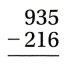Estimate:
930 – 210 = 720
Explanation:
Estimate is a value that is close enough to the right answer,
Since 719 is close to the estimate of 720, the answer is reasonable.

Question 3.
Estimate: __________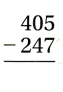Estimate:
400 – 250 = 150
Explanation:
Estimate is a value that is close enough to the right answer,
Since 158 is close to the estimate of 150, the answer is reasonable.

Use the table for 4-6.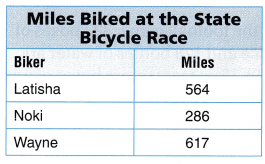Question 4.
About how many more miles did Wayne bike than Latisha?
53 miles
Explanation:
Wayne biked = 617 miles
Latisha biked = 564 miles
By comparing both Wayne biked more.
617 – 564 = 53 miles

Question 5.
Suppose another biker rides 690 miles. How many more miles would this be than the number of miles Noki biked?
404 miles
Explanation:
Suppose another biker rides 690 miles
Noki biked = 286 miles
By comparing both Noki biked less miles.
690 – 286 = 404

Question 6.
How many fewer miles did Wayne bike than Latisha and Noki bike together?
233 miles
Latisha biked = 564 miles
Noki biked = 286 miles
Wayne biked = 617 miles
By combining both Latisha and Noki
564 + 286 = 850
Compare between Wayne and the other two
850 – 617 = 233

Problem Solving

Question 7.
A train travels a distance of 748 miles. Then it travels another 342 miles. How many more miles does the train travel on the first part of the trip?
406 miles
Explanation:
A train travels a distance of 748 miles.
Then it travels another 342 miles.
Number of more miles does the train travel on the first part of the trip
748 – 342 = 406

Question 8.
Denzel wants to subtract 517 – 183. How can he combine the place values to find the difference?
Explanation:
Instead of borrowing or regrouping the number for subtracting,
one can combine the place values.
To combine the place values first combine the tens and ones place.
Then subtract to find the difference.
517 – 183 = 334
Lesson Check

Question 9.
A farm stand has a total of 463 bushels of corn and 284 baskets of apples. How many fewer baskets of apples are there than bushels of corn?
(A) 189
(B) 279
(C) 747
(D) 179
Option (D)
Explanation:
A farm stand has a total of 463 bushels of corn and
number of fewer baskets of apples are there than bushels of corns
463 – 284 = 179

Question 10.
On Saturday a farm stand sells 189 pumpkins. If the farm had a total of 540 pumpkins, how many pumpkins does it still need to sell?
(A) 361
(B) 351
(C) 451
(D) 729
Explanation:
On Saturday a farm stand sells 189 pumpkins.
If the farm had a total of 540 pumpkins,
Number of pumpkins does it still need to sell
540 – 189 = 351

Question 11.
At the football game, 327 boxes of juice and 128 bottles of water are sold. How many more boxes of juice were sold than bottles of water?
(A) 99
(B) 199
(C) 455
(D) 299
Option (B)
Explanation:
Number of juice boxes = 327
Number of water bottles = 128
Number of more boxes of juice were sold than bottles of water bottles
327 – 128 = 199

Question 12.
Rosa has saved 473 pennies. She needs 800 pennies to fill the penny jar. How many more pennies does Rosa need to save?
(A) 327
(B) 337
(C) 427
(D) 273
Option (A)
Explanation:
Rosa has saved 473 pennies.
She needs 800 pennies to fill the penny jar.
more pennies does Rosa need to save
800 – 473 = 327

Question 13.
Multi-Step Courtney has 213 baseball cards. Suri has 162 more cards than Courtney. Ed has 114 fewer cards than Suri. How many cards does Ed have?
(A) 375
(B) 276
(C) 487
(D) 261
Option (D)
Explanation:
Courtney has 213 baseball cards.
Suri has 162 more cards than Courtney.
Ed has 114 fewer cards than Suri.
Number of cards does Ed have
suri has 213 + 162 = 375
Ed has 375 – 114 = 261

Question 14.
Multi-Step Ben collects 213 cans for recycling. Sanjaya collects 189 cans. They want to collect a total of 650 cans to win a prize. How many more cans do they need to collect?
(A) 248
(B) 402
(C) 461
(D) 258